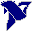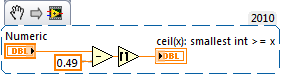# LabVIEW

cancel
Showing results for
Search instead for
Did you mean:

## How to round mathematical (not IEEE)

Hello,

i have a number that should be rounded to the next integer.

I found that there is a IEEE... that described what to do for rounding.

But the mathematic-rounding is different.

The problem is only a x.5 values (1.5 / 2.5 / 3.5 / 4.5).

Labview is rounding to the next even integer - but the correct mathematical way is to round it always up if .5.

How can i do this in Labview?

Thanks for help

Message 1 of 10
(1,507 Views)

## Re: How to round mathematical (not IEEE)

The reason you need IEEE for rounding is that there is no real representation for x.5 in floating point.  You basically always get x.5 +/- epsilon.  If it really matters, you could shift your numbers by machine epsilon.

Message 2 of 10
(1,501 Views)

## Re: How to round mathematical (not IEEE)

Hi Taki,

there is a "real" representation of 0.5 in a floating point mantissa - as it is the same as 1/2 you really only need one bit of accuracy for this...

@OnlyOne:

If you really need to round always upwards:(Any problems because of sign(x) are up to you...)

Best regards,
GerdWusing LV2011SP1 + LV2017 (+LV2020 sometimes) on Win10+cRIO
Message 3 of 10
(1,497 Views)

## Re: How to round mathematical (not IEEE)

@OnlyOne wrote:

Hello,

i have a number that should be rounded to the next integer.

I found that there is a IEEE... that described what to do for rounding.

But the mathematic-rounding is different.

The problem is only a x.5 values (1.5 / 2.5 / 3.5 / 4.5).

Labview is rounding to the next even integer - but the correct mathematical way is to round it always up if .5.

How can i do this in Labview?

Thanks for help

Hi OnlyOne,

It is standard practice to round to the nearest even value when rounding with a 0.5 decimal place, although that's contrary to what I was taught in school! I recently read about this operation in Engineering Mathematics by K.A Stroud, so I'm unsure about whether or not this applies just to engineering. However, I've managed to indirectly derive the functionality you want through this VI below:This method takes away 0.49 from the input double and uses the Round to Positive Infinity VI to round the input values according to your requirements.

I hope that you find this helpful; it'd be nice to find out whether or not this gives you the precision in your values that you're wishing for as well as if we need to find a better implementation!

Message 4 of 10
(1,495 Views)

## Re: How to round mathematical (not IEEE)

hehe, currently i do the same by + 0.1 🙂

I thought there is also a lv-function to round correct in school-style.

Message 5 of 10
(1,488 Views)

## Re: How to round mathematical (not IEEE)

Hi OnlyOne,

"to round correct in school-style."

The problem is: school style is not the same as correct roundingAnd there were reasons to define bankers' rounding in IEEE754 standard!

Best regards,
GerdWusing LV2011SP1 + LV2017 (+LV2020 sometimes) on Win10+cRIO
Message 6 of 10
(1,484 Views)

## Re: How to round mathematical (not IEEE)

But when someone writes 2.5 on paper then it is really a 2.5 and not any epsilon values behind it.

Message 7 of 10
(1,477 Views)

## Re: How to round mathematical (not IEEE)

Hi OnlyOne,

when someone write 2.5 in a computer spreadsheet someone also gets 2.5! (See above.)

The problem starts with any fraction that is not representable by multiples of two!

"But when someone writes ... on paper"

When someone writes on paper he isn't aware of problems arising from rounding errors inherent to number representation on modern computers...

Best regards,
GerdWusing LV2011SP1 + LV2017 (+LV2020 sometimes) on Win10+cRIO
Message 8 of 10
(1,467 Views)

## Re: How to round mathematical (not IEEE)

@GerdW wrote:

Hi Taki,

there is a "real" representation of 0.5 in a floating point mantissa - as it is the same as 1/2 you really only need one bit of accuracy for this...

Indeed, you are right.  Too early in the morning for me to suppress my normal FP accuracy reflex.  🙂

Sorry about the confusion.

Message 9 of 10
(1,463 Views)

## Re: How to round mathematical (not IEEE)

@OnlyOne wrote:

But when someone writes 2.5 on paper then it is really a 2.5 and not any epsilon values behind it.

And computers don't work on paper. What's your point? Computers don't store numbers the way you write them on paper. That's a fact. It's been a fact since computers were invented. You, as the programmer need to understand this and deal with it, or just stick with a paper and pencil. The others here have tried to explain it and offered suggestions. Yet you insist that "school rounding" is the "correct" rounding. There is no correct rounding. There are simply rounding rules. Did you read the link Gerd pointed you to?

Message 10 of 10
(1,458 Views)